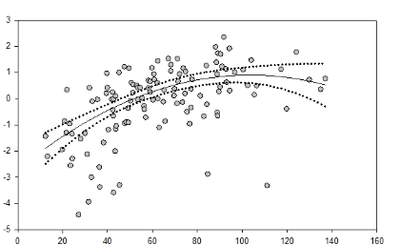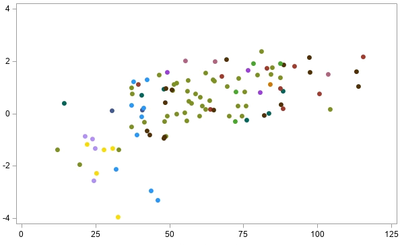## PROC SGPLOT mixing line and scatter plots?

Hi--

I've reached my limit of tinkering to figure this out.

Is there a way to overlay multiple graph types in a single graph?

I have a data set with columns X Y1 Y2 Y3 and Y4.

I want to plot Y1 using a scatter plot (points color coded by group for a little extra level of complication)

I want Y2 to be plotted with just a simple line

I want Y3 and Y4 to be plotted with a different line type

basically a combination of these two styles basically.  I'd like the top one, but with the colors by group as is so easily produced in SGPlot

Thank you.1 ACCEPTED SOLUTION

Accepted Solutions

## Re: PROC SGPLOT mixing line and scatter plots?

Ahh.

proc sgplot data=a;
styleattrs datasymbols=(circlefilled);
scatter y=Y1 x=X1 / group=groupname;
series y=Y2 x=X1;
series y=Y3 x=X1 / lineattrs = (pattern=shortdash);
series y=Y4 x=X1 / lineattrs = (pattern=shortdash);
run;
quit;

3 REPLIES 3

## Re: PROC SGPLOT mixing line and scatter plots?

Yes, in PROC SGPLOT you use the REG command with the option NOMARKERS to get the confidence limits and regression line. Then you add a SCATTER statement to get the data points colored as you wish.

--
Paige Miller

## Re: PROC SGPLOT mixing line and scatter plots?

I saw that feature.  Unfortunately, I'm not using an OLS regression in this case, so the regression methods won't match the models I'm actually building.

I think the solution may lie in the series statements, I'm still tinkering.

## Re: PROC SGPLOT mixing line and scatter plots?

Ahh.

proc sgplot data=a;
styleattrs datasymbols=(circlefilled);
scatter y=Y1 x=X1 / group=groupname;
series y=Y2 x=X1;
series y=Y3 x=X1 / lineattrs = (pattern=shortdash);
series y=Y4 x=X1 / lineattrs = (pattern=shortdash);
run;
quit;

Discussion stats
• 3 replies
• 2333 views
• 0 likes
• 2 in conversation